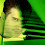## Saturday, October 19, 2013

### Geometry Problem 933: Square, Center, Transversal, Perpendicular, Metric Relations, Congruence

Geometry Problem
Level: Mathematics Education, High School, Honors Geometry, College.

Click on the figure below to enlarge it.1.http://bleaug.free.fr/gogeometry/933.png

bleaug

2.http://www.mathematica.gr/forum/viewtopic.php?f=22&t=37369&start=700#p188400

3.What a wonderful proof!
Thank you, Bleaug

4.thanks for this kind remark...but...
my post does not meet the standards of what can be called a 'proof'. I guess you filled in the gaps yourself. So 50% of the proof is yours ;-)

bleaug

5.Αν Α(0,0), Β(Ο,4),C(4,4),D(4,0) και (ε1)=EO: y-2=λ1(x-2) , (ε2)=FO:y-2=λ2(x-2) τότε

6.<HCO=<BOG=a because <BOC=90. Both triangles HCO and GOB share hypotenuse and the angle a so they are congruent. Similarly OCM and DON are congruent. From those 2 pairs of congruent triangles we have that BG=HO and DN=OM, so the answer is 2(4/sqrt2)^2=16

Ivan Bazarov